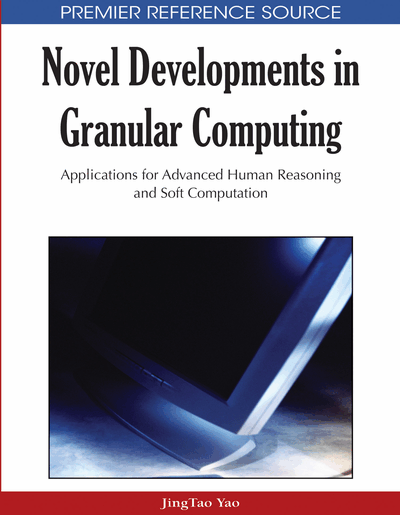# On Characterization of Relation Based Rough Set Algebras

Wei-Zhi Wu, Wen-Xiu Zhang
DOI: 10.4018/978-1-60566-324-1.ch004
OnDemand:
(Individual Chapters)
Available
\$37.50
No Current Special Offers

## Abstract

Rough set theory is one of the most advanced areas popularizing GrC. The basic notions in rough set theory are the lower and upper approximation operators. A rough set algebra is a set algebra with two additional lower and upper approximation operators. In this chapter, we analyze relation based rough set algebras in both crisp and fuzzy environments. We first review the constructive definitions of generalized crisp rough approximation operators, rough fuzzy approximation operators, and fuzzy rough approximation operators. We then present the essential properties of the corresponding lower and upper approximation operators. We also characterize the approximation operators by using the axiomatic approach. Finally, the connection between fuzzy rough set algebras and fuzzy topological spaces is established.
Chapter Preview
Top

## 1. Introduction

Granulation of a universe of discourse is one of the important aspects whose solution has significant bearing on Granular Computing (GrC). Many models and methods of GrC concentrating on concrete models in special contexts have been proposed and studied over the years. Rough set theory is one of the most advanced areas popularizing GrC. The concept of a rough set was originally proposed by Pawlak (1982) as a formal tool for modeling and processing intelligent systems characterized by insufficient and incomplete information. Using the concepts of lower and upper approximations in rough set theory, knowledge hidden in information systems may be unravelled and expressed in the form of decision rules (Pawlak, 1991; Zhang et al, 2003).

The basic operators in rough set theory are approximations. There are at least two approaches to the development of rough set theory, namely the constructive and axiomatic approaches. In the constructive approach, binary relations on a universe of discourse, partitions of the universe of discourse, neighborhood systems, and Boolean algebras are all primitive notions. The lower and upper approximation operators are constructed by means of these notions (Boixader et al., 2000; Dubois & Prade, 1990; Mi et al., 2005; Nanda & Majumda, 1992; Pei, 2005; Pei & Xu, 2004; Pomykala, 1987; Skowron et al., 2003; Slowinski & Vanderpooten, 2000; Wu & Zhang, 2002, 2004; Yao, 1998a, b, c; Yeung et al., 2005; Zhu & Wang, 2007). The constructive approach is suitable for practical applications of rough sets. On the other hand, the axiomatic approach, which is appropriate for studying the structures of rough set algebras, takes the lower and upper approximation operators as primitive notions. In this approach, a set of axioms is used to characterize approximation operators that are the same as the ones produced by using the constructive approach (Lin & Liu, 1994; Liu, 2008); Mi et al., 2008; Mi & Zhang, 2004; Morsi & Yakout, 1998; Radzikowska & Kerre, 2002; Thiele, 2000a, b, 2001a, b; Wu et al., 2003, 2005; Wu & Zhang, 2004; Yang, 2007; Yang & Li, 2006; Yao, 1996, 1998a, b; Yeung et al., 2005). Under this point of view, rough set theory may be interpreted as an extension theory with two additional unary operators. The lower and upper approximation operators are related to the necessity (box) and possibility (diamond) operators in modal logic, the interior and closure operators in topological spaces, and the belief and plausibility functions in Dempster-Shafer theory of evidence (Chuchro, 1993, 1994; Qin & Pei, 2005; Skowron, 1989; Wu et al., 2002; Yao & Lingras, 1998).

## Complete Chapter List

Search this Book:
Reset